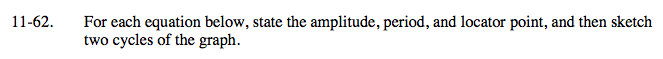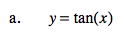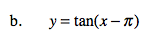Home > A2C > Chapter 11 > Lesson 11.2.2 > Problem11-62

11-62.
1. For each equation below, state the amplitude, period, and locator point, and then sketch two cycles of the graph. Homework Help ✎

1. y = tan(x)

2. y = tan(x − π)Amplitude: N/A
Period: π
Locator point: (0,0)The graph is shifted π radians to the right. How does this affect all of the properties of the graph listed above?